xStatic pressureEncyclopedia
In fluid mechanics
Fluid mechanics
Fluid mechanics is the study of fluids and the forces on them. Fluid mechanics can be divided into fluid statics, the study of fluids at rest; fluid kinematics, the study of fluids in motion; and fluid dynamics, the study of the effect of forces on fluid motion...

the term static pressure has several uses:
• In the design and operation of aircraft
Aircraft
An aircraft is a vehicle that is able to fly by gaining support from the air, or, in general, the atmosphere of a planet. An aircraft counters the force of gravity by using either static lift or by using the dynamic lift of an airfoil, or in a few cases the downward thrust from jet engines.Although...

, static pressure is the air pressure in the aircraft’s static pressure system.
• In fluid dynamics
Fluid dynamics
In physics, fluid dynamics is a sub-discipline of fluid mechanics that deals with fluid flow—the natural science of fluids in motion. It has several subdisciplines itself, including aerodynamics and hydrodynamics...

, static pressure is the pressure
Pressure
Pressure is the force per unit area applied in a direction perpendicular to the surface of an object. Gauge pressure is the pressure relative to the local atmospheric or ambient pressure.- Definition :...

at a nominated point in a fluid. Many authors use the term static pressure in place of pressure to avoid ambiguity.
• The term static pressure is also used by some authors in fluid statics
Fluid statics
Fluid statics is the science of fluids at rest, and is a sub-field within fluid mechanics. The term usually refers to the mathematical treatment of the subject. It embraces the study of the conditions under which fluids are at rest in stable equilibrium...

.

## Static pressure in design and operation of aircraft

An aircraft’s altimeter
Altimeter
An altimeter is an instrument used to measure the altitude of an object above a fixed level. The measurement of altitude is called altimetry, which is related to the term bathymetry, the measurement of depth underwater.-Pressure altimeter:...

is operated by the static pressure system. An aircraft’s airspeed indicator
Airspeed indicator
The airspeed indicator or airspeed gauge is an instrument used in an aircraft to display the craft's airspeed, typically in knots, to the pilot.- Use :...

is operated by the static pressure system and the pitot pressure system
.

The static pressure system is open to the exterior of the aircraft to sense the pressure of the atmosphere at the altitude at which the aircraft is flying. This small opening is called the static port. In flight the air pressure is slightly different at different positions around the exterior of the aircraft. The aircraft designer must select the position of the static port carefully. There is no position on the exterior of an aircraft at which the air pressure, for all angles of attack
Angle of attack
Angle of attack is a term used in fluid dynamics to describe the angle between a reference line on a lifting body and the vector representing the relative motion between the lifting body and the fluid through which it is moving...

, is identical to the atmospheric pressure at the altitude at which the aircraft is flying. The difference in pressure causes a small error in the altitude indicated on the altimeter, and the airspeed
Airspeed
Airspeed is the speed of an aircraft relative to the air. Among the common conventions for qualifying airspeed are: indicated airspeed , calibrated airspeed , true airspeed , equivalent airspeed and density airspeed....

indicated on the airspeed indicator. This error in indicated altitude and airspeed is called position error
Position error
Position error is one of the errors affecting the systems in an aircraft for measuring airspeed and altitude. It is not practical or necessary for an aircraft to have an airspeed indicating system and an altitude indicating system that are exactly accurate...

.
When selecting the position for the static port, the aircraft designer’s objective is to ensure the pressure in the aircraft’s static pressure system is as close as possible to the atmospheric pressure at the altitude at which the aircraft is flying, across the operating range of weight and airspeed. Many authors describe the atmospheric pressure at the altitude at which the aircraft is flying as the freestream
Freestream
The freestream is the air far upstream of an aerodynamic body, that is, before the body has a chance to deflect, slow down or compress the air. Freestream conditions are usually denoted with a \infty symbol, e.g. V_\infty, meaning the freestream velocity...

static pressure
. At least one author takes a different approach in order to avoid a need for the expression freestream static pressure. Gracey has written "The static pressure is the atmospheric pressure at the flight level of the aircraft".
Gracey then refers to the air pressure at any point close to the aircraft as the local static pressure.

## Static pressure in fluid dynamics

The concept of pressure
Pressure
Pressure is the force per unit area applied in a direction perpendicular to the surface of an object. Gauge pressure is the pressure relative to the local atmospheric or ambient pressure.- Definition :...

is central to the study of fluids. A pressure can be identified for every point in a body of fluid, regardless of whether the fluid is in motion or not. Pressure can be measured
Pressure measurement
Many techniques have been developed for the measurement of pressure and vacuum. Instruments used to measure pressure are called pressure gauges or vacuum gauges....

using an aneroid, Bourdon tube, mercury column, or various other methods.

The concepts of total pressure and dynamic pressure arise from Bernoulli's equation and are significant in the study of all fluid flows. (These two pressures are not pressures in the usual sense - they cannot be measured using an aneroid, Bourdon tube or mercury column.) To avoid potential ambiguity when referring to pressure
Pressure
Pressure is the force per unit area applied in a direction perpendicular to the surface of an object. Gauge pressure is the pressure relative to the local atmospheric or ambient pressure.- Definition :...

in fluid dynamics, many authors use the term static pressure to distinguish it from total pressure and dynamic pressure. Static pressure is identical to pressure and can be identified for every point in a fluid flow field.

In Aerodynamics, L.J. Clancy writes: "To distinguish it from the total and dynamic pressures, the actual pressure of the fluid, which is associated not with its motion but with its state, is often referred to as the static pressure, but where the term pressure alone is used it refers to this static pressure."

Bernoulli's equation is fundamental to the dynamics of incompressible fluids. In many fluid flow situations of interest, changes in elevation are insignificant and can be ignored. With this simplification, Bernoulli’s equation for incompressible flows can be expressed as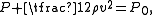where:
•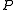is static pressure,
•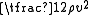is dynamic pressure, usually denoted by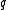,
•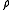is the density
Density
The mass density or density of a material is defined as its mass per unit volume. The symbol most often used for density is ρ . In some cases , density is also defined as its weight per unit volume; although, this quantity is more properly called specific weight...

of the fluid,
•is the flow velocity
Flow velocity
In fluid dynamics the flow velocity, or velocity field, of a fluid is a vector field which is used to mathematically describe the motion of a fluid...

, and
•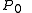is total pressure which is constant along any streamline.

Every point in a steadily flowing fluid, regardless of the fluid speed at that point, has its own static pressure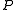, dynamic pressure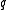, and total pressure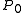. Static pressure and dynamic pressure are likely to vary significantly throughout the fluid but total pressure is constant along each streamline. In irrotational flow, total pressure is the same on all streamlines and is therefore constant throughout the flow.

The simplified form of Bernoulli's equation can be summarised in the following memorable word equation:
static pressure + dynamic pressure = total pressure.

This simplified form of Bernoulli’s equation is fundamental to an understanding of the design and operation of ships, low speed aircraft, and airspeed indicators for low speed aircraft – that is aircraft whose maximum speed will be less than about 30% of the speed of sound
Speed of sound
The speed of sound is the distance travelled during a unit of time by a sound wave propagating through an elastic medium. In dry air at , the speed of sound is . This is , or about one kilometer in three seconds or approximately one mile in five seconds....

.

As a consequence of the widespread understanding of the term static pressure in relation to Bernoulli’s equation, many authors

in the field of fluid dynamics also use static pressure rather than pressure in applications not directly related to Bernoulli’s equation.

The British Standards Institution, in its Standard Glossary of Aeronautical Terms, gives the following definition:
4412 Static pressure The pressure at a point on a body moving with the fluid.

## Static pressure in fluid statics

The term static pressure is sometimes used in fluid statics
Fluid statics
Fluid statics is the science of fluids at rest, and is a sub-field within fluid mechanics. The term usually refers to the mathematical treatment of the subject. It embraces the study of the conditions under which fluids are at rest in stable equilibrium...

to refer to the pressure
Pressure
Pressure is the force per unit area applied in a direction perpendicular to the surface of an object. Gauge pressure is the pressure relative to the local atmospheric or ambient pressure.- Definition :...

of a fluid at a nominated depth in the fluid. In fluid statics the fluid is stationary everywhere and the concepts of dynamic pressure and total pressure are not applicable. Consequently there is little risk of ambiguity in using the term pressure, but some authors choose to use static pressure in some applications.

• Hydrostatic pressure
• Pascal's law
Pascal's law
In the physical sciences, Pascal's law or the Principle of transmission of fluid-pressure states that "pressure exerted anywhere in a confined incompressible fluid is transmitted equally in all directions throughout the fluid such that the pressure ratio remains the same." The law was established...

• Stagnation pressure
Stagnation pressure
In fluid dynamics, stagnation pressure is the static pressure at a stagnation point in a fluid flow.At a stagnation point the fluid velocity is zero and all kinetic energy has been converted into pressure energy . Stagnation pressure is equal to the sum of the free-stream dynamic pressure and...

• Standard conditions for temperature and pressure
Standard conditions for temperature and pressure
Standard condition for temperature and pressure are standard sets of conditions for experimental measurements established to allow comparisons to be made between different sets of data...